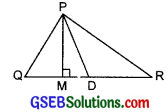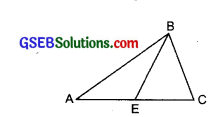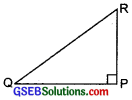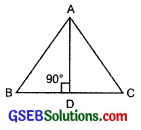# GSEB Solutions Class 7 Maths Chapter 6 The Triangles and Its Properties Ex 6.1

Gujarat Board GSEB Textbook Solutions Class 7 Maths Chapter 6 The Triangles and Its Properties Ex 6.1 Textbook Questions and Answers.

## Gujarat Board Textbook Solutions Class 7 Maths Chapter 6 The Triangles and Its Properties Ex 6.1Question 1.
In ∆PQR, D is the mid-point of $$\overline{Q R}$$.
$$\overline{P M}$$ is _______
PD is _______
Is QM = MR?Solution:
PM is an altitude of ∆PQR.
PD is a median of ∆PQR.
No, QM ≠ MR.Question 2.
Draw rough sketches for the following:
(a) In ∆ABC, BE is a median.
(b) In ∆PQR, PQ and PR are altitudes of the triangle.
(c) In ∆XYZ, YL is an altitude in the exterior of the triangle.
Solution:
(a) In the adjoining figure, $$\overline{B E}$$ is a median of AABC.(b) In right ∆QPR, $$\overline{P Q}$$ and $$\overline{P R}$$ are altitudes of the triangle.(c) In the following figure, $$\overline{Y L}$$ is an altitude of ∆XYZ.Question 3.
Verify by drawing a diagram if the median and altitude of an isosceles triangle can be same. Solution: In the adjoining figure, ∆ABC is an isosceles triangle having AB = AC.
Draw its median AD. Now, with the help of a protractor, measure the ∠ADB.
We find thatThus, $$\overline{A D}$$ is the median as well as an altitude of ∆ABC.